true
true

Lakshiyaa RK# Lakshiyaa RK

## Tutor

22 Students taught

5.0

UrbanPro Rating

Details verified of Lakshiyaa RK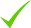IdentityEducation

Know how UrbanPro verifies Tutor details

Identity is verified based on matching the details uploaded by the Tutor with government databases.

## Overview

I can teach every topic of Math & Science in easy manner which makes the student get interested with my extraordinary teaching skills, where student never need to by-heart the content by making them strong on basics.

## Languages Spoken

Telugu

Hindi

English Proficient

## Education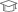SK University 2004

Master of Science (M.Sc.)

## Verified Info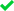Phone VerifiedEmail VerifiedFacebook Verified

## Demo Class

Yes, not charged

Report this Profile

Is this listing inaccurate or duplicate? Any other problem?

Type the letters as shown below *

Please enter the letters as show below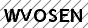## Class 10 Tuition Overview

Class 10 Tuition

Class Location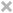Student's HomeTutor's HomeOnline (video chat via skype, google hangout etc)

Years of Experience in Class 10 Tuition

13

Board

International Baccalaureate, CBSE, IGCSE, State, ICSE

IB Subjects taught

Mathematics, Physics, Chemistry, Biology

CBSE Subjects taught

Mathematics, Science

ICSE Subjects taught

Biology, Chemistry, Mathematics, Physics

IGCSE Subjects taught

Physics, Mathematics, Biology, Chemistry

Taught in School or College

Yes

State Syllabus Subjects taught

Science, Mathematics

Teaching Experience in detail in Class 10 Tuition

I have an excellent experience of Teaching Math/Phy/Chem of CBSE Curriculum since 13 years with best track record.

## Reviews (22)

this is test message this is test message this is test message this is test message this is test message this is test message this is test message

5.0 out of 5.0 22 reviews

5.00522
P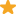"It helped me a lot to improve, he teaches very well math and science. He will be friendly, he takes extra class when exams."

S"It is the best tutorial in which all students are observed carefully and mistakes will be corrected. Unlike any other tutorial in this tutorial concepts will be made clear. "

B"Very good learning atmosphere. Awesome teaching and interactive class.Sir well motivate the students. Though sir is strict he is friendly too. "

H"It helped me in all the ways especially in maths and science. Sir will refer different authors books for extra knowledge. There is I. D system for the safety of students our Brain starts thinking Mentally and physically how to solve the sums. It will help in all the sums and each sum will be solved by us and special classes for IIT foundation will really help us in competitive exams and higher classes if we will not understand sir will explain the sum again. "

Have you attended any class with Lakshiyaa ? Write a Review

## FAQs

1. Which school boards of Class 10 do you teach for?

International Baccalaureate, CBSE, IGCSE and others

2. Do you have any prior teaching experience?

Yes

3. Which classes do you teach?

I teach Class 10 Tuition, Class 6 Tuition, Class 7 Tuition, Class 8 Tuition and Class 9 Tuition Classes.

4. Do you provide a demo class?

Yes, I provide a free demo class.

5. How many years of experience do you have?

I have been teaching for 13 years.

Answered on 08/11/2018 Tuition/Class VI-VIII Tuition/Mathematics

Let the no. of stamps with Ankush be 'x' Then the no. of stamps with Ram = (4/5) x = 4x / 5 As the total stamps together = 4500 No. Of stamps with (Ankush+ Ram) = 4500 x + 4x/5 = 4500 5x + 4x =... ...more

Let the no. of stamps with Ankush be 'x'

Then the no. of stamps with Ram = (4/5) x = 4x / 5

As the total stamps together = 4500

No. Of stamps with (Ankush+ Ram) = 4500

x + 4x/5 = 4500

5x + 4x = 5 × 4500

9x = 5 × 4500

x = 5×4500/9

x = 5×500

No. Of stamps with Ankush = 2500

Dislike Bookmark

Answer - (A) Electric Current ( I ) = Quantity of Charge (Q) time (t) Charge Q = ne (no. of electrons electrons × charge on electron ) Charge on electron = 1.6 × 10-19 C here n = 5 ×1016 t = 1min... ...more

Electric Current ( I ) = Quantity of Charge (Q)

time (t)

Charge Q = ne    (no. of electrons electrons × charge on electron )

Charge on electron = 1.6 × 10-19 C

here n = 5 ×1016      t = 1min 20sec = 80 sec

I =  ne   = 5 ×1016 × 1.6 × 10-19   = 1016 × 10-19  = 10-4 A = 103 mA = 10-1mA = 0.1mA

t                     80                              10                             104       ( 1A = 103mA )

Dislike Bookmark

in above si units of charge is C -coulomb
Dislike Bookmark

D) since Ampere.Sec is the Unit of Charge. Explanation: Electric Current(I) = Rate of charge flow I = Q(Charge) / t (time) SI units of Current = C / sec = ampere now inn C/sec = amp send sec other side then C = amp.sec so C for si unit of Charge that means units of charge is amp.sec ...more

D) since Ampere.Sec is the Unit of Charge.

Explanation: Electric Current(I) = Rate of charge flow

I = Q(Charge) / t (time)

SI units of Current = C / sec = ampere

now inn C/sec = amp

send sec other side

then C = amp.sec

so C for si unit of Charge

that means units of charge is amp.sec

Dislike Bookmark

As we know the triangle, parallelogram standing on the same side and between same parallel lines then ar(Triangle) = 1/2.ar(parallelogram) and ar(parallagoram) = 2, ar(triangle) = 2 (15) cm.sq ar of Parallelogram ABPD = 30cm.sq
Dislike Bookmark

Lakshiyaa Directions

x
Class 10 Tuition

Class LocationStudent's HomeTutor's HomeOnline (video chat via skype, google hangout etc)

Years of Experience in Class 10 Tuition

13

Board

International Baccalaureate, CBSE, IGCSE, State, ICSE

IB Subjects taught

Mathematics, Physics, Chemistry, Biology

CBSE Subjects taught

Mathematics, Science

ICSE Subjects taught

Biology, Chemistry, Mathematics, Physics

IGCSE Subjects taught

Physics, Mathematics, Biology, Chemistry

Taught in School or College

Yes

State Syllabus Subjects taught

Science, Mathematics

Teaching Experience in detail in Class 10 Tuition

I have an excellent experience of Teaching Math/Phy/Chem of CBSE Curriculum since 13 years with best track record.

Class 9 Tuition

Class LocationStudent's HomeTutor's HomeOnline (video chat via skype, google hangout etc)

Years of Experience in Class 9 Tuition

13

Board

International Baccalaureate, CBSE, IGCSE, State, ICSE

IB Subjects taught

Mathematics, Physics, Chemistry, Biology

CBSE Subjects taught

Mathematics, Science

ICSE Subjects taught

Biology, Chemistry, Mathematics, Physics

IGCSE Subjects taught

Physics, Mathematics, Biology, Chemistry

Taught in School or College

Yes

State Syllabus Subjects taught

Science, Mathematics

Class 6 Tuition

Class LocationStudent's HomeTutor's HomeOnline (video chat via skype, google hangout etc)

Years of Experience in Class 6 Tuition

13

Board

CBSE, ICSE, State, IGCSE, International Baccalaureate

IB Subjects taught

Mathematics, Science

CBSE Subjects taught

Mathematics, Science

ICSE Subjects taught

Mathematics, Physics

IGCSE Subjects taught

Science, Mathematics

Taught in School or College

Yes

State Syllabus Subjects taught

Science, Mathematics

Class 7 Tuition

Class LocationStudent's HomeTutor's HomeOnline (video chat via skype, google hangout etc)

Years of Experience in Class 7 Tuition

13

Board

CBSE, ICSE, State, IGCSE, International Baccalaureate

IB Subjects taught

Mathematics, Science

CBSE Subjects taught

Mathematics, Science

ICSE Subjects taught

Mathematics, Physics

IGCSE Subjects taught

Science, Mathematics

Taught in School or College

Yes

State Syllabus Subjects taught

Science, Mathematics

Class 8 Tuition 4.7

Class LocationStudent's HomeTutor's HomeOnline (video chat via skype, google hangout etc)

Years of Experience in Class 8 Tuition

13

Board

CBSE, ICSE, State, IGCSE, International Baccalaureate

IB Subjects taught

Mathematics, Science

CBSE Subjects taught

Mathematics, Science

ICSE Subjects taught

Mathematics, Physics

IGCSE Subjects taught

Science, Mathematics

Taught in School or College

Yes

State Syllabus Subjects taught

Science, Mathematics

Answered on 08/11/2018 Tuition/Class VI-VIII Tuition/Mathematics

Let the no. of stamps with Ankush be 'x' Then the no. of stamps with Ram = (4/5) x = 4x / 5 As the total stamps together = 4500 No. Of stamps with (Ankush+ Ram) = 4500 x + 4x/5 = 4500 5x + 4x =... ...more

Let the no. of stamps with Ankush be 'x'

Then the no. of stamps with Ram = (4/5) x = 4x / 5

As the total stamps together = 4500

No. Of stamps with (Ankush+ Ram) = 4500

x + 4x/5 = 4500

5x + 4x = 5 × 4500

9x = 5 × 4500

x = 5×4500/9

x = 5×500

No. Of stamps with Ankush = 2500

Dislike Bookmark

Answer - (A) Electric Current ( I ) = Quantity of Charge (Q) time (t) Charge Q = ne (no. of electrons electrons × charge on electron ) Charge on electron = 1.6 × 10-19 C here n = 5 ×1016 t = 1min... ...more

Electric Current ( I ) = Quantity of Charge (Q)

time (t)

Charge Q = ne    (no. of electrons electrons × charge on electron )

Charge on electron = 1.6 × 10-19 C

here n = 5 ×1016      t = 1min 20sec = 80 sec

I =  ne   = 5 ×1016 × 1.6 × 10-19   = 1016 × 10-19  = 10-4 A = 103 mA = 10-1mA = 0.1mA

t                     80                              10                             104       ( 1A = 103mA )

Dislike Bookmark

in above si units of charge is C -coulomb
Dislike Bookmark

D) since Ampere.Sec is the Unit of Charge. Explanation: Electric Current(I) = Rate of charge flow I = Q(Charge) / t (time) SI units of Current = C / sec = ampere now inn C/sec = amp send sec other side then C = amp.sec so C for si unit of Charge that means units of charge is amp.sec ...more

D) since Ampere.Sec is the Unit of Charge.

Explanation: Electric Current(I) = Rate of charge flow

I = Q(Charge) / t (time)

SI units of Current = C / sec = ampere

now inn C/sec = amp

send sec other side

then C = amp.sec

so C for si unit of Charge

that means units of charge is amp.sec

Dislike Bookmark

As we know the triangle, parallelogram standing on the same side and between same parallel lines then ar(Triangle) = 1/2.ar(parallelogram) and ar(parallagoram) = 2, ar(triangle) = 2 (15) cm.sq ar of Parallelogram ABPD = 30cm.sq
Dislike Bookmark

Lakshiyaa RK describes himself as Tutor. He conducts classes in Class 10 Tuition, Class 6 Tuition and Class 7 Tuition. Lakshiyaa is located in Zaheerabad, Raichur. Lakshiyaa takes Online Classes- via online medium. He has 13 years of teaching experience . Lakshiyaa has completed Master of Science (M.Sc.) from SK University in 2004. He is well versed in Kannada, Telugu, English and Hindi. Lakshiyaa has got 22 reviews till now with 100% positive feedback.

Recommended Profiles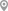Raichur, RaichurRaichur Jawahar Nagar, RaichurAndroon Quilla Ghalib Nagar, RaichurRaichur Jawahar Nagar, RaichurRaichur Saath Katchery, RaichurRaichur, Raichur

X

Let us shortlist and give the best tutors and institutes.

or

Send Enquiry to Lakshiyaa

Let Lakshiyaa know you are interested in their class